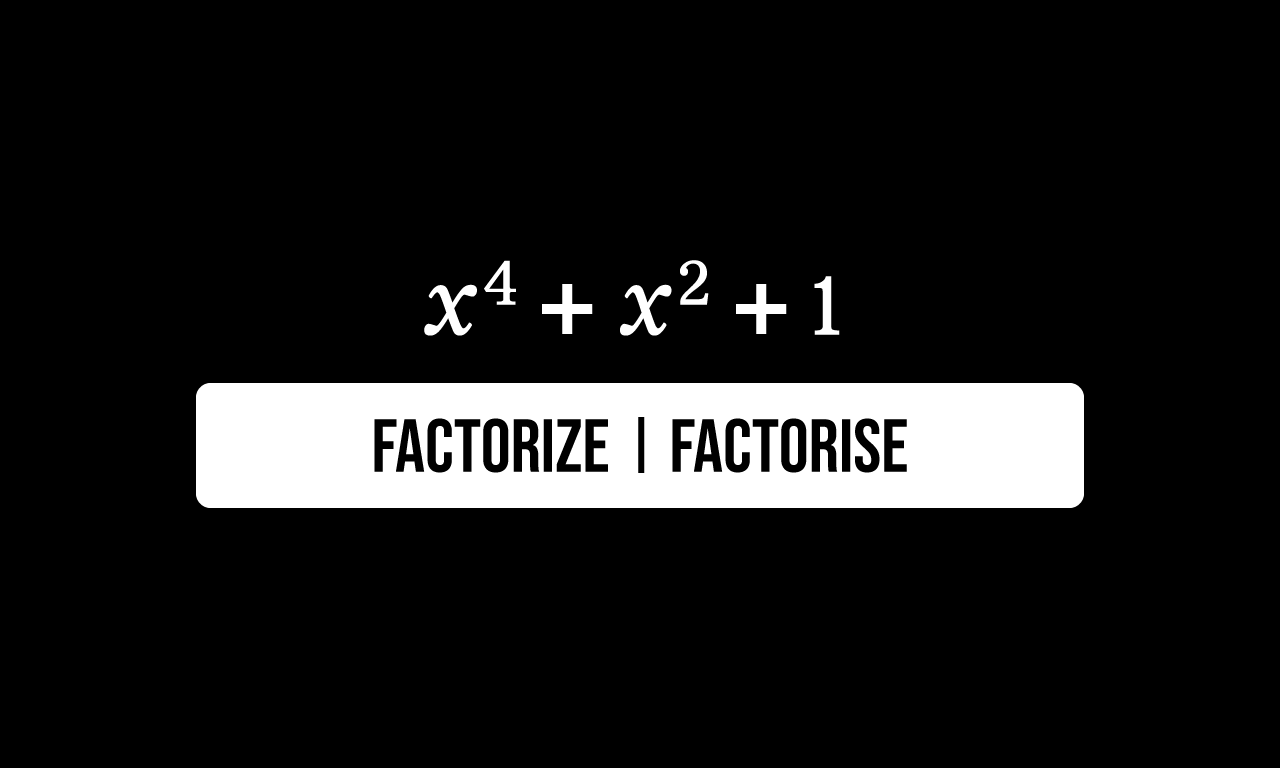# Factorize $x^4+x^2+1$

$x$ is a variable in this problem and a polynomial is defined in terms of $x$ as follows.$x^4+x^2+1$

The algebraic expression is basically a trinomial, which means the expression consists of three terms. One term is $x$ raised to the power of $4$, second term is $x$ squared and the third term is a constant term $1$. The addition of three of them forms this special algebraic expression.

The algebraic expression should be factored in this algebraic problem. So, let’s learn how to factorize the expression in algebraic form.

### Prepare the expression for factorization

As per the commutative property of addition, the given algebraic expression can be written as follows.

$\implies$ $x^4+x^2+1$ $\,=\,$ $x^4+1+x^2$

$=\,\,\,$ $x^4+1+x^2$

The $x$ raised to the fourth power creates problem for factorizing the above polynomial because we studied only the polynomials, which consists of squares and cubes. Hence, we should convert the term the $4$th power of $x$ into either square or cube form.

$=\,\,\,$ $x^{2 \times 2}+1+x^2$

According to the power rule of exponents, the $x$ raised to the power of the product of two $2$s can be written as a square of $x$ squared.

$=\,\,\,$ $\big(x^2\big)^2+1+x^2$

Now, let’s denote the $x$ squared by a variable $y$ for our convenience, which means take $y \,=\, x^2$. Now, convert the whole expression in terms of $y$ by replacing $x$ squared by its assumed value.

$=\,\,\,$ $(y)^2+1+y$

$=\,\,\,$ $y^2+1+y$

The first term is a term in square form. The second term is a constant term and it can be expressed in square form too. This technique allows us to factorise the given mathematical expression in algebraic form.

$=\,\,\,$ $y^2+(1 \times 1)+y$

$=\,\,\,$ $y^2+(1^2)+y$

$=\,\,\,$ $y^2+1^2+y$

### Transform the expression in square form

The first two terms are in square form and it requires an additional term $2y$ for converting the part of the expression in square form of a binomial. So, add a term $2y$ to the expression and subtract the expression by the same term to maintain the equilibrium of the mathematical expression.

$=\,\,\,$ $y^2+1^2+2y-2y+y$

$=\,\,\,$ $y^2+1^2+2y-y$

The first three terms in the above expression represents the expansion of the square of sum of two terms rule. So, the three terms can be simplified as a square of the binomial $y+1$.

$=\,\,\,$ $(y+1)^2-y$

The above mathematical expression is simplified in terms but the given algebraic expression is defined in $x$. So, replace the value of $y$ in $x$ in the above mathematical expression.

$=\,\,\,$ $\big(x^2+1\big)^2-x^2$

### Factorize the Difference of the squares

Let’s represent the algebraic expression $x^2+1$ by a variable $z$ for our convenience, which means assume $z \,=\, x^2+1$. Then, the algebraic expression can be written in terms of $z$ as follows.

$\implies$ $\big(x^2+1\big)^2-x^2$ $\,=\,$ $(z)^2-x^2$

$=\,\,\,$ $z^2-x^2$

The mathematical expression expresses the difference of squares. So, it can be factored as a product of two binomials.

$=\,\,\,$ $(z+x)(z-x)$

Now, replace the value of $z$ by its actual value in terms of $x$.

$=\,\,\,$ $(x^2+1+x)(x^2+1-x)$

$=\,\,\,$ $(x^2+x+1)(x^2-x+1)$

Latest Math Topics
Jun 26, 2023
Jun 23, 2023

###### Math Questions

The math problems with solutions to learn how to solve a problem.

Learn solutions

Practice now

###### Math Videos

The math videos tutorials with visual graphics to learn every concept.

Watch now

###### Subscribe us

Get the latest math updates from the Math Doubts by subscribing us.